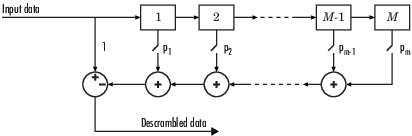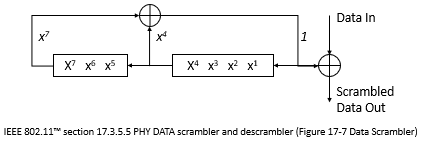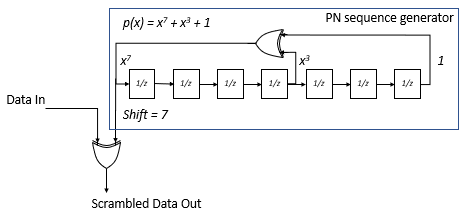# comm.Descrambler

Descramble input signal

## Description

The `comm.Descrambler` System object™ applies multiplicative descrambling to input data. It performs the inverse operation of the `comm.Scrambler` object used in the transmitter.

This schematic shows the multiplicative descrambler operation. The adders and subtracter operate modulo N, where N is the value specified by the CalculationBase property.At each time step, the input causes the contents of the registers to shift sequentially. Using the Polynomial property, you specify the on or off state for each switch in the descrambler. To make the `comm.Descrambler` object reverse the operation of the `comm.Scrambler` object, use the same property settings in both objects. If there is no signal delay between the scrambler and the descrambler, then the InitialConditions in the two objects must be the same.

Note

To apply additive descrambling to input data, you can use the `comm.PNSequence` System object and the `xor` function. For an example, see Additive Scrambling of Input Data.

To descramble an input signal:

1. Create the `comm.Descrambler` object and set its properties.

2. Call the object with arguments, as if it were a function.

## Creation

### Syntax

``descrambler = comm.Descrambler``
``descrambler = comm.Descrambler(base,poly,cond)``
``descrambler = comm.Descrambler(___,Name,Value)``

### Description

````descrambler = comm.Descrambler` creates a descrambler System object. This object descrambles the input data by using a linear feedback shift register that you specify with the Polynomial property.```

example

````descrambler = comm.Descrambler(base,poly,cond)` creates the descrambler object with the CalculationBase property set to `base`, the Polynomial property set to `poly`, and the InitialConditions property set to `cond`.Example: ```comm.Descrambler(8,'1 + x^-2 + x^-3 + x^-5 + x^-7',[0 3 2 2 5 1 7])``` sets the calculation base to `8`, and the descrambler polynomial and initial conditions as specified.```

example

````descrambler = comm.Descrambler(___,Name,Value)` sets properties using one or more name-value pairs and either of the previous syntaxes. Enclose each property name in single quotes.Example: `comm.Descrambler('CalculationBase',2)````

## Properties

expand all

Unless otherwise indicated, properties are nontunable, which means you cannot change their values after calling the object. Objects lock when you call them, and the `release` function unlocks them.

If a property is tunable, you can change its value at any time.

Range of input data used in the descrambler for modulo operations, specified as a nonnegative integer. The input and output of this object are integers from `0` to `CalculationBase``1`.

Data Types: `double`

Connections for linear feedback shift registers in the descrambler, specified as a character vector, integer vector, or binary vector. The `Polynomial` property defines if each switch in the descrambler is on or off. Specify the polynomial as:

• A character vector, such as `'1 + x^-6 + x^-8'`. For more details on specifying polynomials in this way, see Representation of Polynomials in Communications Toolbox.

• An integer vector, such as `[0 -6 -8]`, listing the descrambler coefficients in order of descending powers of x-1, where p(x-1) = 1 + p1x-1 + p2x-2 + ...

• A binary vector, such as `[1 0 0 0 0 0 1 0 1]`, listing the powers of x that appear in the polynomial that have a coefficient of 1. In this case, the order of the descramble polynomial is one less than the binary vector length.

Example: `'1 + x^-6 + x^-8'`, `[0 -6 -8]`, and `[1 0 0 0 0 0 1 0 1]` all represent this polynomial:

p(x-1) = 1 + x-6 + x-8

Data Types: `double` | `char`

• `'Property'` – Specify descrambler initial conditions by using the InitialConditions property.

• `'Input port'` – Specify descrambler initial conditions by using an additional input argument, initcond, when calling the object.

Data Types: `char`

Initial conditions of descrambler registers when the simulation starts, specified as a nonnegative integer vector. The length of `InitialConditions` must equal the order of the Polynomial property. The vector element values must be integers from `0` to CalculationBase`1`.

#### Dependencies

This property is available when InitialConditionsSource is set to `'Property'`.

Descrambler state reset port, specified as `false` or `true`. If `ResetInputPort` is `true`, you can reset the descrambler object by using an additional input argument, reset, when calling the object.

#### Dependencies

This property is available when InitialConditionsSource is set to `'Property'`.

## Usage

### Syntax

``descrambledOut = descrambler(signal)``
``descrambledOut = descrambler(signal,initcond)``
``descrambledOut = descrambler(signal,reset)``

### Description

example

````descrambledOut = descrambler(signal)` descrambles the input signal. The output is the same data type and length as the input vector. ```

example

````descrambledOut = descrambler(signal,initcond)`provides an additional input with values specifying the initial conditions of the linear feedback shift register.This syntax applies when you set the InitialConditionsSource property of the object to `'Input port'`.```
````descrambledOut = descrambler(signal,reset)` provides an additional input indicating whether to reset the state of the descrambler.This syntax applies when you set the InitialConditionsSource property of the object to `'Property'` and the ResetInputPort to `true`.```

### Input Arguments

expand all

Input signal, specified as a column vector.

Example: `descrambledOut = descrambler([0 1 1 0 1 0])`

Data Types: `double` | `logical` | `int8` | `int16` | `int32` | `uint8` | `uint16` | `uint32`

Initial descrambler register conditions when the simulation starts, specified as a nonnegative integer column vector. The length of `initcond` must equal the order of the Polynomial property. The vector element values must be integers from `0` to CalculationBase`1`.

Example: `descrambledOut = descrambler(signal,[0 1 1 0])` corresponds to possible initial register states for a descrambler with a polynomial order of `4` and a calculation base of `2` or higher.

Data Types: `double`

Reset initial state of the descrambler when the simulation starts, specified as a scalar. When the value of `reset` is nonzero, the object is reset before it is called.

Example: `descrambledOut = descrambler(signal,0)` descrambles the input signal without resetting the descrambler states.

Data Types: `double`

### Output Arguments

expand all

Descrambled output, returned as a column vector with the same data type and length as signal.

## Object Functions

To use an object function, specify the System object as the first input argument. For example, to release system resources of a System object named `obj`, use this syntax:

`release(obj)`

expand all

 `step` Run System object algorithm `release` Release resources and allow changes to System object property values and input characteristics `reset` Reset internal states of System object

## Examples

collapse all

Scramble and descramble 8-ary data using `comm.Scrambler` and `comm.Descrambler` System objects™ having a calculation base of 8.

Create scrambler and descrambler objects, specifying the calculation base, polynomial, and initial conditions using input arguments. The scrambler and descrambler polynomials are specified with different but equivalent data formats.

```N = 8; scrambler = comm.Scrambler(N,'1 + x^-2 + x^-3 + x^-5 + x^-7', ... [0 3 2 2 5 1 7]); descrambler = comm.Descrambler(N,[1 0 1 1 0 1 0 1], ... [0 3 2 2 5 1 7]);```

Scramble and descramble random integers. Display the original data, scrambled data, and descrambled data sequences.

```data = randi([0 N-1],5,1); scrData = scrambler(data); deScrData = descrambler(scrData); [data scrData deScrData]```
```ans = 5×3 6 7 6 7 5 7 1 7 1 7 0 7 5 3 5 ```

Verify that the descrambled data matches the original data.

`isequal(data,deScrData)`
```ans = logical 1 ```

Scramble and descramble quaternary data while changing the initial conditions between function calls.

Create scrambler and descrambler System objects having a calculation base of 4. Set the `InitialConditionsSource` property to `'Input port'` so you can set the initial conditions as an argument to the object.

```N = 4; scrambler = comm.Scrambler( ... N,'1 + z^-3', ... 'InitialConditionsSource','Input port'); descrambler = comm.Descrambler( ... N,'1 + z^-3', ... 'InitialConditionsSource','Input port');```

Preallocate memory for the error vector which will be used to store errors output by the `symerr` function.

`errVec = zeros(10,1);`

Scramble and descramble random integers while changing the initial conditions, `initCond`, each time the loop executes. Use the `symerr` function to determine if the scrambling and descrambling operations result in symbol errors.

```for k = 1:10 initCond = randperm(3)'; data = randi([0 N-1],5,1); scrData = scrambler(data,initCond); deScrData = descrambler(scrData,initCond); errVec(k) = symerr(data,deScrData); end```

Examine `errVec` to verify that the output from the descrambler matches the original data.

`errVec`
```errVec = 10×1 0 0 0 0 0 0 0 0 0 0 ```

Digital communications systems commonly use additive scrambling to randomize input data to aid in timing synchronization and power spectral requirements. The `comm.Scrambler` System object™ implements multiplicative scrambling but does not support additive scrambling. To perform additive scrambling you can use the `comm.PNSequence` System object. This example implements the additive scrambling specified in IEEE 802.11™ by scrambling input data with an output sequence generated by the comm.PNSequence System object. For a Simulink® model that implements a similar workflow, see the Additive Scrambling of Input Data in Simulink example.

This figure shows an additive scrambler, that uses the generator polynomial ${\mathit{x}}^{7}+{\mathit{x}}^{4}+1$, as specified in figure 17-7 of IEEE 802.11 Section 17.3.5.5 .Comparing the shift register specified in 802.11 with the shift register implementated using a comm.PNSequence System object, note that the two shift register schematics are mirror images of each other. Therefore, when configuring the comm.PNSequence System object to implement an additive scrambler, you must reverse values for the generator polynomial, the initial states, and the mask output. To take the output of the register from the leading end, specify a shift value of 7.For more information about the 802.11 scrambler, see  and the `wlanScramble` (WLAN Toolbox) reference page.

Define variables for the generator polynomial, shift value for the output, an initial shift register state, a frame of input data, and a variable containing the 127-bit scrambler sequence specified in section 17.3.5.5 of the IEEE 802.11 standard. Create a PN sequence object that initializes the registers by using an input argument.

```genPoly = 'x^7 + x^3 + 1'; % Generator polynomial shift = 7; % Shift value for output spf = 127; % Samples per frame initState = [1 1 1 1 1 1 1]; % Initial shift register state dataIn = randi([0 1],spf,1); ieee802_11_scram_seq = logical([ ... 0 0 0 0 1 1 1 0 1 1 1 1 0 0 1 0 1 1 0 0 1 ... 0 0 1 0 0 0 0 0 0 1 0 0 0 1 0 0 1 1 0 0 0 ... 1 0 1 1 1 0 1 0 1 1 0 1 1 0 0 0 0 0 1 1 0 ... 0 1 1 0 1 0 1 0 0 1 1 1 0 0 1 1 1 1 0 1 1 ... 0 1 0 0 0 0 1 0 1 0 1 0 1 1 1 1 1 0 1 0 0 ... 1 0 1 0 0 0 1 1 0 1 1 1 0 0 0 1 1 1 1 1 1 1])'; pnSeq = comm.PNSequence( ... Polynomial=genPoly, ... InitialConditionsSource="Input Port", ... Mask=shift, ... SamplesPerFrame=spf, ... OutputDataType="logical"); pnsequence = pnSeq(initState);```

Compare the PN sequence object output to the IEEE 802.11 127-bit scrambler sequence to confirm the generated PN sequence matches the 802.11 specified sequence.

`isequal(ieee802_11_scram_seq,pnsequence)`
```ans = logical 1 ```

Scramble input data according to the 802.11 specified additive scrambler by modulo-adding input data with the PN sequence output.

`scrambledOut = xor(dataIn,pnSeq(initState));`

Descramble the scrambled data by applying the same scrambler and initial conditions to the scrambled data.

`descrambledData = xor(scrambledOut,pnSeq(initState));`

Verify that the descrambled data matches the input data.

`isequal(dataIn,descrambledData)`
```ans = logical 1 ```

Reference

 IEEE Std 802.11™-2020 (Revision of IEEE Std 802.11™-2016). "Part 11: Wireless LAN Medium Access Control (MAC) and Physical Layer (PHY) Specifications." IEEE Standard for Information technology — Telecommunications and information exchange between systems. Local and metropolitan area networks — Specific requirements.

## Version History

Introduced in R2012a